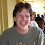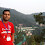## Sunday, August 23, 2015

### Interesting properties of the number 24 on someone's 24th wedding anniversary

The story you are about to read is true. Only the names have been changed to protect the innocent. The Alice and Bob below are not the crypto Alice and Bob.

------------------------------------------------------
BOB (to ALICE): Its our 24th anniversary! Last year when it was our 23rd anniversary we celebrated by having you tell me that 23 was the ONLY number that required 9 cubes so sum to it, and that its open how many cubes you need for large n, though its between 4 and 7. Oh that was fun! What do you have planned for our 24th anniversary!

ALICE (to BOB): I've prepared FIVE facts about 24! Oh, I mean 24, not 24 factorial! We'll see which one you want to discuss.  Here they are:

1) 24 is the largest nat number n such that all nat numbers m ≤  sqrt;(n)   m divides n.

2) 24 is the least nat number that has exactly 8 distinct factors. (1,2,3,4,6,8,12,24)

3) 24 is the ONLY number m≥2 such that 1^2 + 2^2 + ... + m^2 is a square. Its 70^2, so if we are married 70 years, I'll have an interesting fact about 24.

4) Let S be an n-sphere. How many spheres of the same size as S can kiss S? Thats the kissing number, called kiss(n).  kiss(2)=6 (so given a circle you can position 6 identical circles that kiss it), kiss(3)=12 (thats 3-dim), and kiss(4)=24.

5) Its one of the few numbers that is the title of a TV show.

BOB:  Since its our anniversary I'll go with the kissing number~ Mathematically I'd go with the square thing.
---------------------------------------------------

I am sure that for all numbers ≤ 94 one can come up with some facts of interest. The book Those Fascinating Number has an interesting fact about many numbers. The least number that it has no interesting fact about is 95, but I suspect Alice and Bob won't be married that long.

What are the coolest numbers (mathematically)? See here  for a possible answer.

1.24 is also one of the most enjoyable math games I know. Wikipedia describes it as a card game, but I generally just play it with whichever four numbers are available.
https://en.wikipedia.org/wiki/24_Game

2.Point number 3 is not clear exactly.
Does this means adding the squares upto 24^2 ?

1.(This is bill gasarch)
Yes: a^2 means a squared.
1^2 + 2^2 + 3^2 + 4^2 + 5^2 + ... + 24^2 = 70^2

3.It is their last factorial anniversary!

4.I had asked a question on MathOverflow about the existence of "boring" numbers, which are numbers that don't satisfy a Diophantine equation with small coefficients and degree. It was closed for being vague, but I suspect that something can be done with the idea.

5."The least number that it has no interesting fact about is 95,"

How interesting. It is the first number where the only thing interesting about it is there is nothing interesting about it.

1.And this generalizes to the following result:

Thm
All natural numbers are interesting.

Pf.
We proceed by contradiction. Let S be the set of non-interesting natural numbers. By the well ordering-principle it follows that S has a minimum element, call it n. Thus n is the smallest number for which there is nothing interesting. This makes n interesting, a contradiction.
qed

6.It looks like 239 also requires 9 cubes: https://projecteuclid.org/euclid.bams/1183502007

7.We were married on the 24th because it's 4 factorial. And we made a bigger deal of our 24th anniversary than our 25th. Although, you got my wife's name wrong; it's Liz, not Alice.

8.24 is my birth date! I also wish to get get married on the same